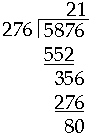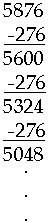Division starts from left - why? Name: Salil Dave Who is asking: Parent Level: All Question: Addition, subtraction and multiplications start with right most digit and proceed left, but division starts from left-most digits and goes right ... why? Hi, You could start division on the right but the procedure (algorithm) we usually use, that starts from the left, is more efficient. For example if I want to divide 5876 by 276 using our normal algorithm, the way I learned it, I would write:This procedure has two steps. First you see that 276 divides into 5876 twenty times with a remainder of 356. Secondly 276 divides into 356 once with a remainder of 80. Thus, in total, 276 divides into 5876 twenty-one times with a remainder of 80. Working from the right would be repeated subtraction.Repeat subtracting 276 until the remainder is less than 276. We know from the above that we can do that twenty-one times and end with a remainder of 80. The advantage of the algorithm that works from the left is that in one step you see that 276 divides into 5876 at least twenty times. I hope this helps, Harley Go to Math Central